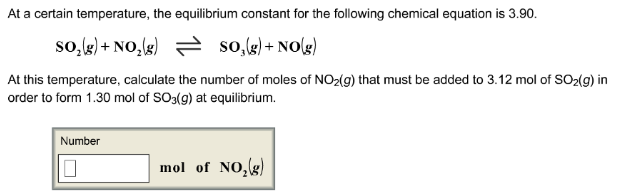# Problem: At a certain temperature, the equilibrium constant for the following chemical equation is 3.90. SO2 (g) + NO2 (g) ⇌ SO3 (g) + NO (g) At this temperature, calculate the number of moles of NO2(g) that must be added to 3.12 mol of SO2(g) in order to form 1.30 mol of SO3(g) at equilibrium.

###### FREE Expert Solution
82% (252 ratings)###### Problem Details

At a certain temperature, the equilibrium constant for the following chemical equation is 3.90.

SO(g) + NO2 (g) ⇌ SO(g) + NO (g)

At this temperature, calculate the number of moles of NO2(g) that must be added to 3.12 mol of SO2(g) in order to form 1.30 mol of SO3(g) at equilibrium.